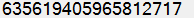# Date & Time

Should be enabled the MSBuild support.

In examples below, we use the MSBuild Property Functions and you can use any static method or property of these system classes:

## Format & Culture

Sortable format: ~ `yyyy/MM/dd`, e.g.: `2016/08/21` for InvariantCulture

For specific culture, use for example:

``````\$([System.DateTime]::Parse("21.08.2016", '\$([System.Globalization.CultureInfo]::GetCultureInfo("ru-RU"))'))
``````
``````\$([System.DateTime]::Parse("08/21/2016", '\$([System.Globalization.CultureInfo]::GetCultureInfo("en-US"))'))
``````

## How to get the number of ticks from specific date

We can use the next methods:

for example:

``````\$([System.DateTime]::Parse("2015/02/17").ToBinary())
``````
``````\$([System.DateTime]::Parse("2015/02/17 07:21").ToBinary())
``````

### Number of ticks from current date and time

We also should use the next properties:

for example:

``````\$([System.DateTime]::UtcNow.Ticks)
``````

## How to get the total Minutes or Hours from Ticks

You should use the TimeSpan Properties:

and TimeSpan.FromTicks method, for example:

``````\$([System.TimeSpan]::FromTicks(635618792404338780).TotalHours)
\$([System.TimeSpan]::FromTicks(635618792404338780).TotalMinutes)
``````

You also can use the ToString(string) method to get an integer value, e.g.:

``````\$([System.TimeSpan]::FromTicks(635618792404338780).TotalHours.ToString("0"))
\$([System.TimeSpan]::FromTicks(635618792404338780).TotalMinutes.ToString("0"))
``````

### How to get delta between the time (Ticks)

• Total minutes from Ticks 1 to Ticks 2:
``````\$([System.TimeSpan]::FromTicks(\$([MSBuild]::Subtract(635618821282084745, 635618792404338780))).TotalMinutes.ToString("0"))
``````
• Total seconds from Ticks 1 to Ticks 2:
``````\$([System.TimeSpan]::FromTicks(\$([MSBuild]::Subtract(635618821282084745, 635618792404338780))).TotalSeconds.ToString("0"))
``````

## Custom Date and Time Format Strings

MSDN

``````\$([System.DateTime]::UtcNow.ToString("yyyy.MM.dd_HH;mm;ss.ffff"))
``````

Result: `2016.02.07_10;56;54.8265`

## Standard Date and Time Format Strings

MSDN

``````\$([System.DateTime]::UtcNow.ToString("o"))
``````

Result: `2016-02-07T10:57:59.4937445Z`

``````\$([System.DateTime]::UtcNow.ToString("R"))
``````

Result: `Sun, 07 Feb 2016 10:59:03 GMT`

## Full example for build number

You can try this If needed a some additional build number and similar.

Notes:

• If you plan use the TotalMinutes as base of rev. you should note that's correct way only if you have one or less assemblies for 1 minute. Otherwise, you should use the similar TotalSeconds, but again only if you have one or less assemblies for 1 second etc.
• Give preference to UTC (Coordinated Universal Time) However! it does not give any warranty for unique numbers. Please remember about this, and be careful for developing in team - for this case use the our CI features and others…
• Use our Wizard as an easy step for quick results.